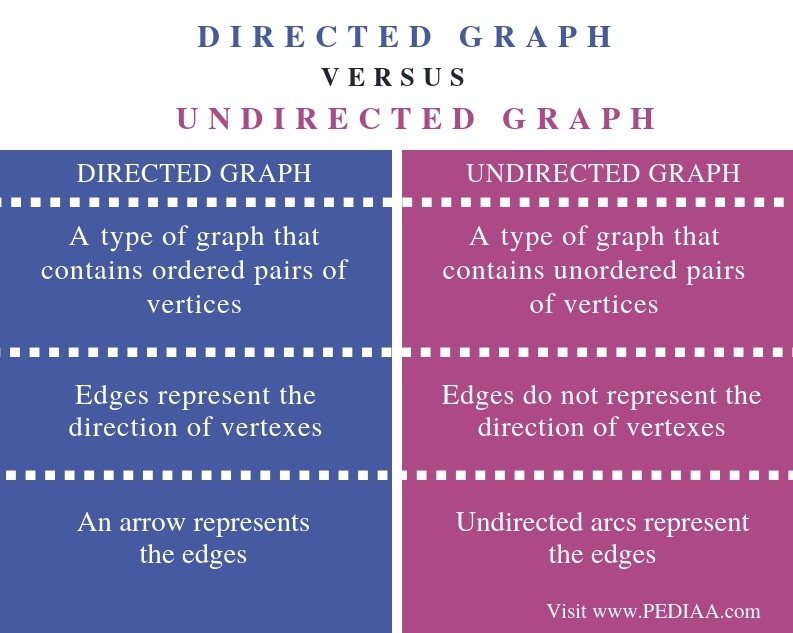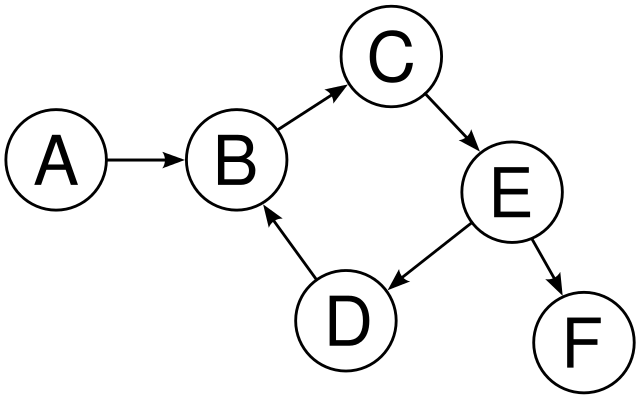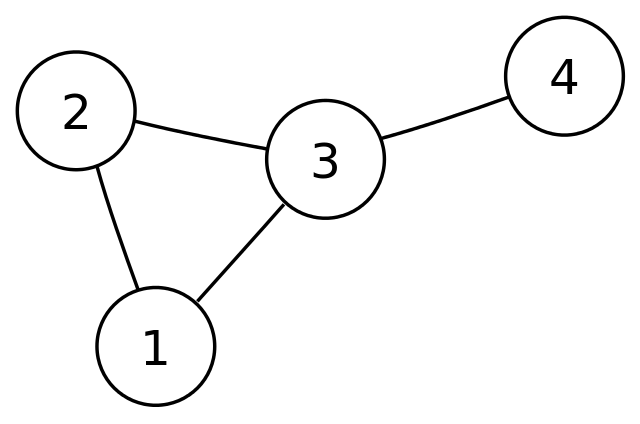# What is the Difference Between Directed and Undirected Graph

The main difference between directed and undirected graph is that a directed graph contains an ordered pair of vertices whereas an undirected graph contains an unordered pair of vertices.

A graph is a nonlinear data structure that represents a pictorial structure of a set of objects that are connected by links. A graph represents data as a network. Two major components in a graph are vertex and edge. A vertex is a data element while an edge is a link that helps to connect vertices. There are mainly two types of graphs as directed and undirected graphs.

### Key Areas Covered

1. What is Directed Graph
– Definition, Functionality
2. What is Undirected Graph
– Definition, Functionality
3. What is the Difference Between Directed and Undirected Graph
– Comparison of Key Differences

Key Terms

Directed Graph, Graph, Nonlinear Data Structure, Undirected Graph## What is Directed Graph

When a graph has an ordered pair of vertexes, it is called a directed graph. The edges of the graph represent a specific direction from one vertex to another. When there is an edge representation as (V1, V2), the direction is from V1 to V2. The first element V1 is the initial node or the start vertex. The second element V2 is the terminal node or the end vertex.In the above graph, vertex A connects to vertex B. A is the initial node and node B is the terminal node. The direction is from A to B. Therefore; we cannot consider B to A direction. Similarly, vertex D connects to vertex B. D is the initial node while B is the terminal node. The direction is from D to B, and we cannot consider B to D. Likewise, the connected vertexes have specific directions.

Set of vertices (V) – {A, B, C, D, E, F}

Set of edges (E) – {(A,B),(B,C),(C,E),(E,D),(D,E),(E,F)}

## What is Undirected Graph

When a graph has an unordered pair of vertexes, it is an undirected graph. In other words, there is no specific direction to represent the edges. The vertexes connect together by undirected arcs, which are edges without arrows. If there is an edge between vertex A and vertex B, it is possible to traverse from B to A, or A to B as there is no specific direction.Above is an undirected graph. There is no direction in any of the edges. It is possible to traverse from 2 to 3, 3 to 2, 1 to 3, 3 to 1 etc.

Set of vertices (V) – {1, 2, 3, 4}

Set of edges (E) – {(1, 2), (2, 1), (2, 3), (3, 2), (1, 3), (3, 1), (3, 4), (4, 3)}

## Difference Between Directed and Undirected Graph

### Definition

A directed graph is a type of graph that contains ordered pairs of vertices while an undirected graph is a type of graph that contains unordered pairs of vertices. Thus, this is the main difference between directed and undirected graph.

### Direction

Furthermore, in directed graphs, the edges represent the direction of vertexes. However, in undirected graphs, the edges do not represent the direction of vertexes. Hence, this is another difference between directed and undirected graph.

### Representation

Moreover, the symbol of representation is a major difference between directed and undirected graph. In directed graphs, arrows represent the edges, while in undirected graphs, undirected arcs represent the edges.

### Conclusion

There are two types of graphs as directed and undirected graphs. The main difference between directed and undirected graph is that a directed graph contains an ordered pair of vertices whereas an undirected graph contains an unordered pair of vertices.

##### Reference:

1. “Graphs in Data Structure”, Data Flow Architecture, Available here.
2. “DS Graph – Javatpoint.” Www.javatpoint.com, Available here.

##### Image Courtesy:

1. “Directed graph, cyclic” By David W. at German Wikipedia.(Original text: David W.) – Transferred from de.wikipedia to Commons. Transfer was stated to be made by User:Ddxc (Public Domain) via Commons Wikimedia
2. “Undirected graph” By No machine-readable author provided. Luks assumed (based on copyright claims) – Own work assumed (based on copyright claims) (Public Domain) via Commons Wikimedia